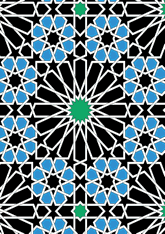# Zellij dado, Sa'di tombes, Marrakeshdata166/P76

## Geometry

• The symmetry group of the tiling is *442 (p4m).
• All the internal angles of the constituent polygons are a multiple of 22.5°.
• Contains two regular 8-pointed star polygons with vertex angle of 90°.
• Contains one regular 16-pointed star polygon with vertex angle of 45°.
• There are 8 non-regular reflective tiles (including 2 kites).
• The tiling satisfies the interlace condition and has one finite interlace and one infinite interlace with straight cross-overs.
• The tiling is edge-to-edge.
• As drawn, contains about 451 polygons.

## References

Publications referenced: